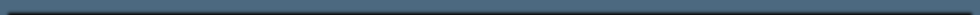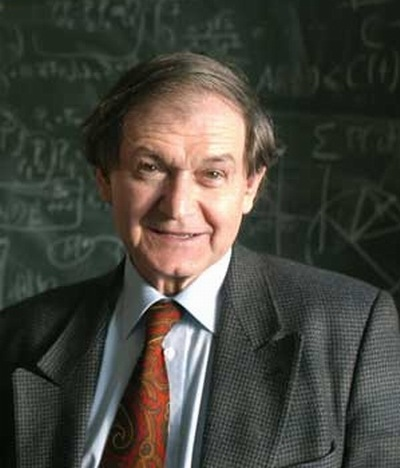# Understanding Matter“The contemporary understanding of material is very different now from the way it used to be. If we consider what matter really is, we now understand it as much more of a mathematical thing… But I think that matter itself is now much more of a mental substance…”

–Roger Penrose (English Mathematician, Theoretical Physicist and Emeritus Rouse Ball Professor of Mathematics at the Mathematical Institute, University of Oxford, 1931-)